# Types, theory of

(diff) ← Older revision | Latest revision (diff) | Newer revision → (diff)

A formal first-order theory (cf. Formal system), one variant of which — the simple theory of types — is described below. The terminology "theory of types" has no rigidly fixed meaning. It denotes formal theories which are distinguished in the following way. First, there are linguistic tools available in the formal system for expressing relationships described in words as: "the sequence x1…xn belongs to y" or "y is performed on x1…xn" ; let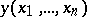denote the expression in quotation marks. Secondly, there is the decomposition of the object domain into strata, or types, forming a hierarchy of types (not necessarily linear, and not necessarily countable), and the presence of type-theoretic comprehension axioms (or their equivalents). If variables running through the objects of typeare denoted by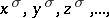then type-theoretical comprehension axioms have the form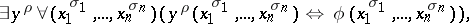(*)

where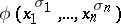is a formula relative to the system with free variables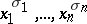, and the typeof the variable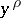must belong (this is the main point of type-theoretic systems) to a higher level in the hierarchy of types than the types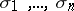. The typeis usually uniquely determined by the types. It is denoted by. Thus, in a type-theoretic system a propertyand objectswhich satisfy this property belong to different strata. One often adds an extensionality axiom, which identifies properties with equal content. In this case the type-theoretic system can be regarded as a set-theoretic system, since it satisfies the principle that "a set is completely determined by its elements" .

Type-theoretic systems were introduced by B. Russell in connection with his discovery of a contradiction in set theory. Putting a set and its elements at different levels leads to a point of view regarding antinomies (cf. Antinomy) according to which the appearance of a contradiction is explained by the non-predicative nature of some set-theoretical definitions. Here a definition of some object is called non-predicative if the object itself takes part in the definition, or, what amounts to the same thing, if the definition makes no sense without assuming in advance the existence of the object. Thus, in (*), regarded as a definition of the object, the non-predicativity has not been completely removed since, in the formula, there may appear quantifiers in variables which run through the same domain that the objectbelongs to. Hence one considers also predicative type-theoretic systems, in which one carries out a further decomposition of the object domains. In such systems,in (*) must belong to a domain distinct from the domains run through by the bound variables in.

One considers type-theoretic systems in which the types are ordered as some initial segment of the ordinal numbers or as the set of (positive and negative) integers, and also systems in which all formulas are objects of a well-defined type, and systems which allow expressions of infinite length (or the means of substituting such expressions, for example quantifiers over an index type). Logics of the second and higher orders may be regarded as type-theoretic systems.

## The simple theory of types.

The language of the simple theory of types consists of: for each natural number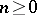, variables of type,; the two-place predicate symbol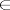; the logical connectives and quantifiers,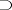,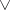,,,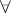,; and the brackets ( and ).

The formulas of the simple theory of types are constructed in the usual inductively-defined way: the expressions of the formare formulas; ifandare formulas andis a variable, then,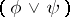,,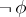,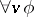,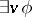are formulas. The notationis used as an abbreviation for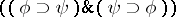.

## Non-logical axioms of the simple theory of types.

A1) The comprehension axiom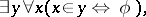whereandare variables of typesand, respectively, andis an arbitrary formula in the language of the simple theory of types not containing the free variable.

A2) The extensionality axiom: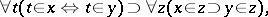whereare variables of types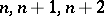, respectively.

A3) The infinity axiom: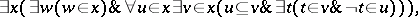whereis a variable of type 2. The types of the remaining variables can be recovered uniquely. Here the notations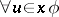and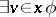denote the formulas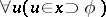and, respectively, and the expression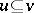is an abbreviation for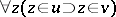.

Logical axioms and inference rules. These are the logical axioms and inference rules (cf. Derivation rule) of the classical predicate calculus, reformulated in the appropriate language. These axioms and rules determine the class of derivable formulas or theorems in the simple theory of types. This class can be defined semantically.

A mathematical structure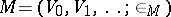, where, is called a model of the simple theory of types if all non-logical axioms of the simple theory of types are satisfied in it. Gödel's completeness theorem for the predicate calculus implies that the class of theorems for the simple theory of types coincides with the class of formulas which are true in all models of the simple theory of types. The simple theory of types is a fairly strong theory. Within it one can imbed arithmetic and analysis (that is, second-order arithmetic), and consider the initial ordinal numbers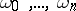, whereis any natural number given in advance. It has a simple intuitive set-theoretic model. For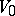one can take any infinite set, and each subsequent levelconsists of the subsets of the previous level. The relation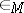is the natural one. The existence of a set-theoretic model can be used for a formal proof of the consistency of the simple theory of types in the framework of a sufficiently powerful axiomatic theory. Such a consistency proof can be constructed, for example, within the Zermelo system, which is obtained from the Zermelo–Fraenkel systemby omitting the axiom of substitution, but retaining the axiom of separation.